# Whether the given curve has vertical asymptotes or horizontal asymptotes.### Single Variable Calculus: Concepts...

4th Edition
James Stewart
Publisher: Cengage Learning
ISBN: 9781337687805### Single Variable Calculus: Concepts...

4th Edition
James Stewart
Publisher: Cengage Learning
ISBN: 9781337687805

#### Solutions

Chapter 2, Problem 6RCC

(a)

To determine

## To find: Whether the given curve has vertical asymptotes or horizontal asymptotes.

Expert Solution

The given curve has no asymptotes.

### Explanation of Solution

Result used:

Definition of vertical asymptote:

The vertical asymptote of the function y=f(x) is x=a, if y approaches ± as x approaches a from the right or left.

Limit is defined as limxaf(x)=±(or)limxa+f(x)=± (or) both.

Definition of horizontal asymptote:

The horizontal asymptote of the function y=f(x) is y=b, if y approaches b as x approaches ±.

Limit is defined as limxf(x)=b(or)limx+f(x)=b(or) both.

Graph:

The graph of a function y=x4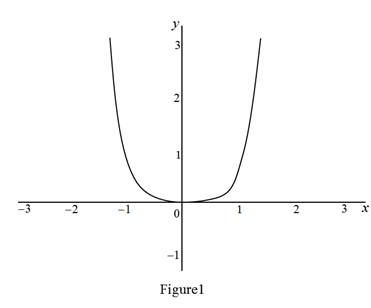Calculation:

For vertical asymptotes:

limx0+f(x)=limx0+x4               =(0)4=0

There are no vertical asymptotes.

For horizontal asymptotes:

limx±f(x)=limx±x4               =(±)4=

There are no horizontal asymptotes.

(b)

To determine

### To find: Whether the curve y = sin x have vertical asymptotes or horizontal asymptotes.

Expert Solution

The graph of y = sin x has no asymptotes.

### Explanation of Solution

The graph of a function y=sin(x)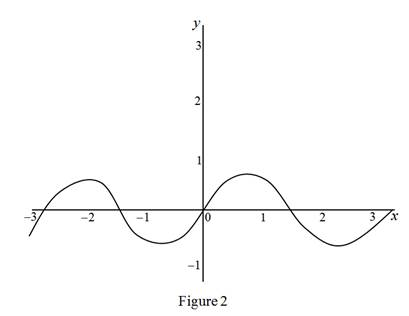There are no vertical asymptotes, because the function y=sin(x) is defined for all real numbers so there are no vertical lines.

For vertical asymptotes:

limx0+f(x)=limx0+sin(x)               =sin(0)=0

There are no vertical asymptotes.

For horizontal asymptotes:

There are no horizontal asymptotes, because from the graph the function y=sin(x) oscillates between –1 and 1 so there are no horizontal lines. The limit of the function is

limx±f(x)=limx±sin(x)limx±f(x)=sin()[1,1]

(c)

To determine

### To find: Whether the curve y = tan x have vertical asymptotes or horizontal asymptotes.

Expert Solution

There are only vertical asymptotes at x=(2n+1)π2.

### Explanation of Solution

Graph:

The graph of a function y=tan(x).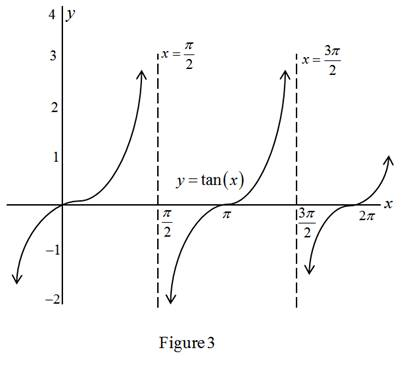Calculation:

For horizontal asymptotes,

limx±f(x)=limx±tan(x)limx±f(x)=tan()

There are no horizontal asymptotes.

From the graph there are vertical asymptotes at x=(2n+1)π2, n = 0,1,2,3,…

(d)

To determine

### To find: Whether the given curve has vertical asymptotes or horizontal asymptotes

Expert Solution

There are only horizontal asymptotes at y=π2 and y=π2.

### Explanation of Solution

Graph:

The graph of a function y=tan1(x).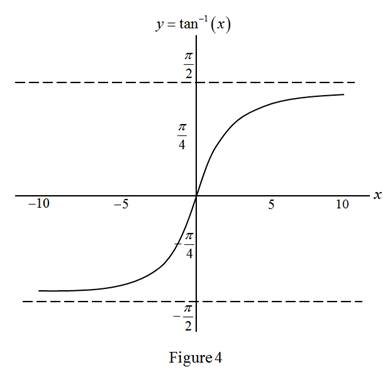Calculation:

For vertical asymptotes,

limx0+f(x)=limx0+tan1(x)               =tan1(0)=0

There are no vertical asymptotes.

From the graph there are horizontal asymptotes at y=π2 and y=π2.

(e)

To determine

### To find: Whether the given curve has vertical asymptotes or horizontal asymptotes

Expert Solution

There are only horizontal asymptote at y=0.

### Explanation of Solution

Graph:

The graph of a function y=ex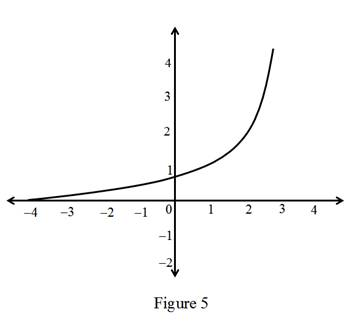Calculation:

For vertical asymptotes,

limx0+f(x)=limx0+ex               =e0=1

There are no vertical asymptotes.

From the graph there are horizontal asymptote at y=0.

(f)

To determine

### To find: Whether the given curve has vertical asymptotes or horizontal asymptotes.

Expert Solution

There are only vertical asymptote at x=0.

### Explanation of Solution

Graph:

The graph of a function y=ln(x)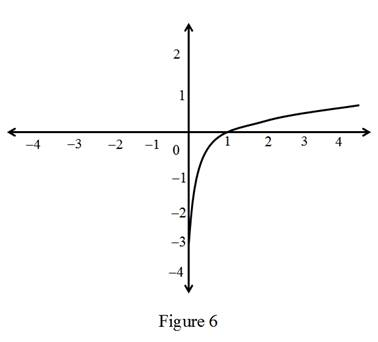Calculation:

For horizontal asymptotes,

limx±f(x)=limx±ln(x)               =ln()=

There are no horizontal asymptotes

From the graph there is vertical asymptote at x=0.

(g)

To determine

### To find: Whether the given curve has vertical asymptotes or horizontal asymptotes

Expert Solution

There is vertical asymptote at x=0.

There is horizontal asymptote at y=0.

### Explanation of Solution

Graph:

The graph of a function y=1x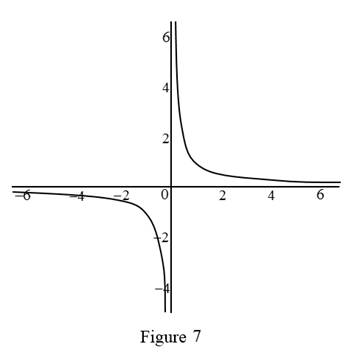Calculation:

There is horizontal asymptote at y=0.

That is limx(1x)=0.

There is vertical asymptote at x=0.

That is limx0+(1x)= and limx0(1x)=.

(h)

To determine

### To find: Whether the given curve has vertical asymptotes or horizontal asymptotes.

Expert Solution

The given curve has no asymptotes.

### Explanation of Solution

Graph:

The graph of a function y=x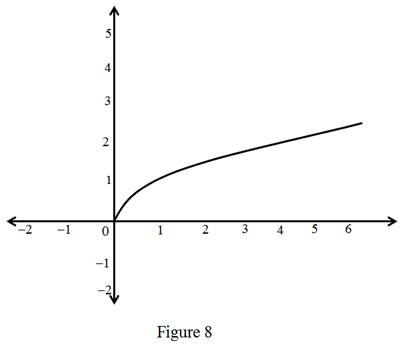Calculation:

For vertical asymptotes,

limx0+f(x)=limx0+x               =0=0

There are no vertical asymptotes.

For horizontal asymptotes:

limx±f(x)=limx±x               ==

There are no horizontal asymptotes.

### Have a homework question?

Subscribe to bartleby learn! Ask subject matter experts 30 homework questions each month. Plus, you’ll have access to millions of step-by-step textbook answers!